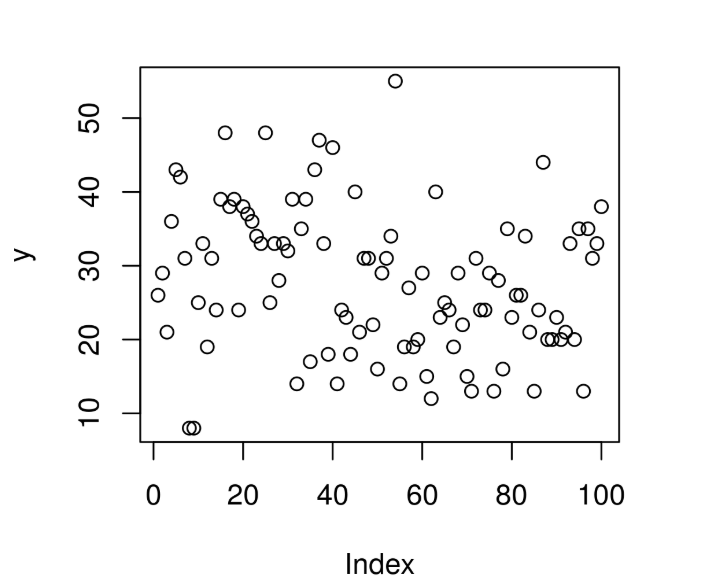# Compute Randomly Drawn Wilcoxon Signedrank Density in R Programming – rsignrank() Function

`rsignrank()` function in R Language is used to compute random density for Wilcoxon Signedrank Statistic Distribution over a sequence of random Numeric values.

Syntax: rsignrank(N, n)

Parameters:
N: Size of Random Vector
n: Sample Size

Example 1:

 `# R Program to compute random ` `# Wilcoxon Signedrank Density ` ` `  `# Setting seed for ` `# random number generation ` `set``.seed(``1000``) ` ` `  `# Set Vector size ` `N <``-` `20` ` `  `# Calling rsignrank() Function ` `y <``-` `rsignrank(N, n ``=` `10``) ` `y `

Output:

```  26 29 21 36 43 42 31  8  8 25 33 19 31 24 39 48 38 39 24 38
```

Example 2:

 `# R Program to compute random ` `# Wilcoxon Signedrank Density ` ` `  `# Setting seed for ` `# random number generation ` `set``.seed(``1000``) ` ` `  `# Set Vector size ` `N <``-` `100` ` `  `# Calling rsignrank() Function ` `y <``-` `rsignrank(N, n ``=` `10``) ` ` `  `# Plot a graph ` `plot(y) `

Output:My Personal Notes arrow_drop_upCheck out this Author's contributed articles.

If you like GeeksforGeeks and would like to contribute, you can also write an article using contribute.geeksforgeeks.org or mail your article to contribute@geeksforgeeks.org. See your article appearing on the GeeksforGeeks main page and help other Geeks.

Please Improve this article if you find anything incorrect by clicking on the "Improve Article" button below.

Article Tags :

Be the First to upvote.

Please write to us at contribute@geeksforgeeks.org to report any issue with the above content.